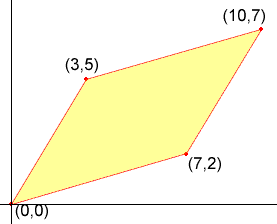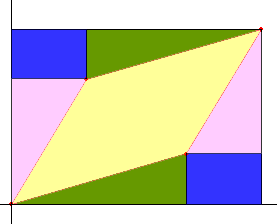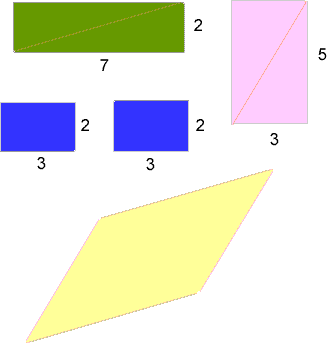Who is asking: Student Level: Secondary Question: Find the area of the parallelogram with vertices (0,0),(7,2),(10,7),(3,5) Hi, There are many ways to approach this problem. Since you haven't given me any indication of what techniques you know, I am going to do it in the way I think is most natural. The technique is scissors and tape. Below is a diagram of the parallelogram.Drop a perpendicular line from (10,7) to the x-axis and another from (10,7) to the y-axis, to form a rectangle that is 10 units by 7 units, and hence has area 70 square units. Now chop this rectangle into pieces by line segments parallel to the axes as in the diagram below.Take your scissors cut along the line segments and then tape the pieces back together as in the diagram below.I now have 4 rectangles and a parallelogram whose areas add to 70 square units. Calculate the areas of the rectangles and subtract their total from 70 to find the area of the parallelogram. This technique may seem elementary but the essential idea that you can find the area of a region by chopping it up and summing the areas of the pieces is exactly what you need when you use calculus to find areas. Cheers, Penny Go to Math Central